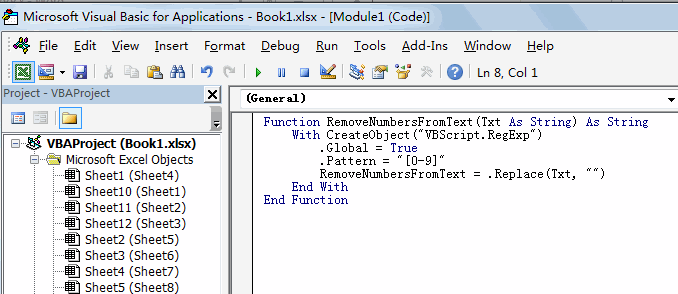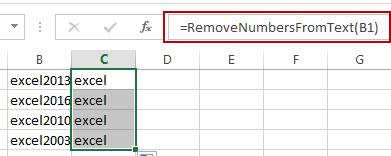# How to Remove Numbers from Text in Excel

This post will guide you how to remove all numbers from a text string in one cell in Excel. How do I strip all numeric characters from a given cell with a formula in Excel. How do I remove numbers from cells with text using a User Defined Function in Excel.

## Remove Numbers from Text Using Formula

Assuming that you have a list of data in range B1:B4 which contain test string values. And you want to look for a formula to remove all numbers from text string in given range of cells. You can use an array formula based on the TEXTJOIN function. Like this:

`=TEXTJOIN("",TRUE,IF(ISERR(MID(B1,ROW(INDIRECT("1:100")),1)+0),MID(B1,ROW(INDIRECT("1:100")),1),""))`

Type this formula into a blank cell and press Ctrl + Shift + Enter keys to change the formula as array formula.

## Remove Numbers from Text Using User Defined Function

You can also write a User Defined Function with VBA code to achieve the same result of removing all numbers from text string in the selected range of cells in Excel. Here are the steps:

#1 open your excel workbook and then click on “Visual Basic” command under DEVELOPER Tab, or just press “ALT+F11” shortcut.#2 then the “Visual Basic Editor” window will appear.

#3 click “Insert” ->”Module” to create a new module.#4 paste the below VBA code into the code window. Then clicking “Save” button.#5 back to the current worksheet, then type the following formula in a blank cell, and then press Enter key.

=RemoveNumbersFromText(B1)

#6 drag the AutoFill handle over other cells to  remove numbers from text in one cell.### Related Functions

• Excel ROW function
The Excel ROW function returns the row number of a cell reference.The ROW function is a build-in function in Microsoft Excel and it is categorized as a Lookup and Reference Function.The syntax of the ROW function is as below:= ROW ([reference])….
• Excel IF function
The Excel IF function perform a logical test to return one value if the condition is TRUE and return another value if the condition is FALSE. The IF function is a build-in function in Microsoft Excel and it is categorized as a Logical Function.The syntax of the IF function is as below:= IF (condition, [true_value], [false_value])….
• Excel MID function
The Excel MID function returns a substring from a text string at the position that you specify.The syntax of the MID function is as below:= MID (text, start_num, num_chars)…
• Excel INDIRECT function
The Excel INDIRECT function returns the cell reference based on a text string, such as: type the text string “A2” in B1 cell, it just a text string, so you can use INDIRECT function to convert text string as cell reference….